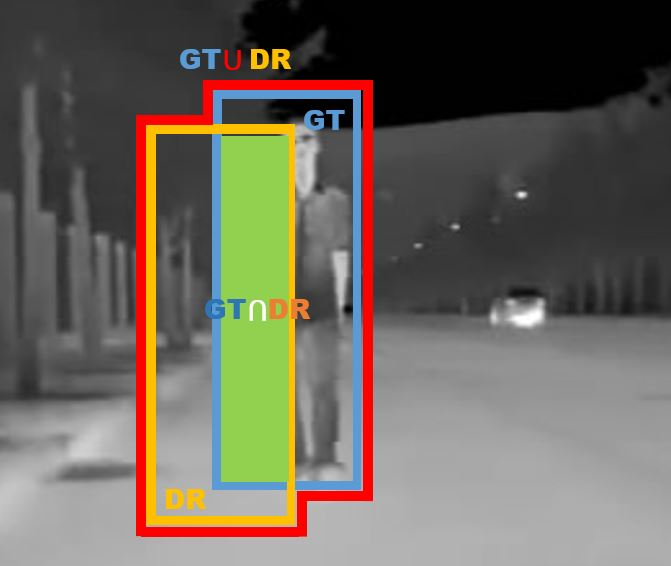# 目标检测（一）目标检测评价指标

## 大雁与飞机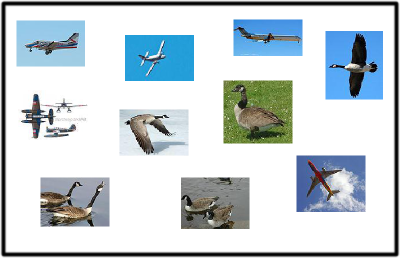True positives : 飞机的图片被正确的识别成了飞机。
True negatives: 大雁的图片没有被识别出来，系统正确地认为它们是大雁。
False positives: 大雁的图片被错误地识别成了飞机。
False negatives: 飞机的图片没有被识别出来，系统错误地认为它们是大雁。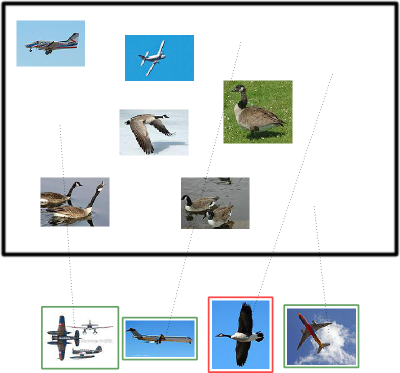True positives : 有三个，画绿色框的飞机。
False positives: 有一个，画红色框的大雁。

True negatives : 有四个，这四个大雁的图片，系统正确地没有把它们识别成飞机。
False negatives: 有两个，两个飞机没有被识别出来，系统错误地认为它们是大雁。

## Precision 与 Recall

Precision其实就是在识别出来的图片中，True positives所占的比率：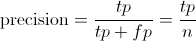Recall 是被正确识别出来的飞机个数与测试集中所有飞机的个数的比值：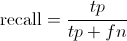Recall的分母是(True positives + False negatives)，这两个值的和，可以理解为一共有多少张飞机的照片。

## 调整阈值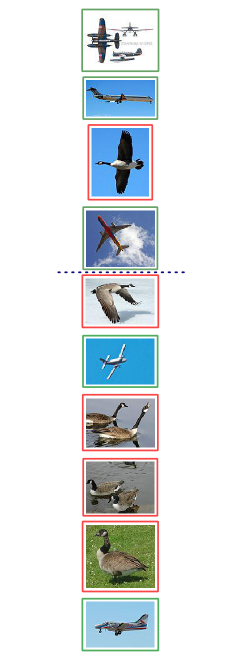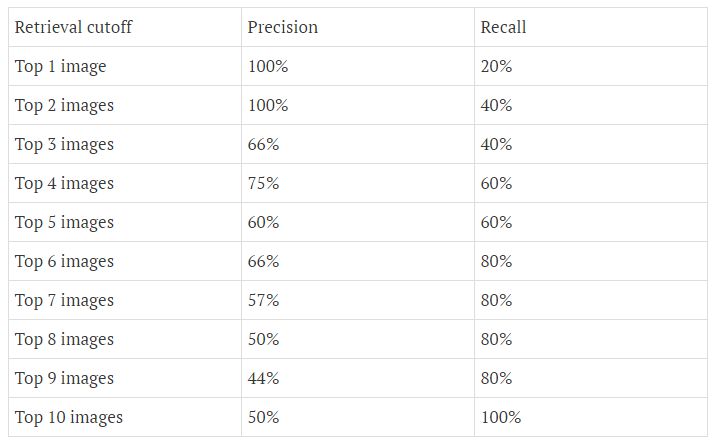## Precision-recall 曲线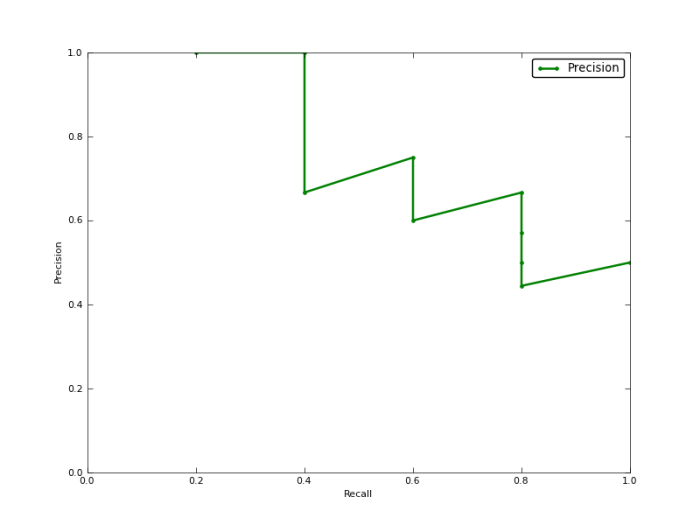## Approximated Average precision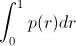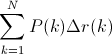=(1 * （0.2-0）) + (1 * (0.4-0.2)) + (0.66 * (0.4-0.4)) + (0.75 * (0.6-0.4)) + (0.6 * (0.6-0.6)) + (0.66 * (0.8-0.6)) + (0.57 * (0.8-0.8)) + (0.5 * (0.8-0.8)) + (0.44 * (0.8-0.8)) + (0.5 * (1-0.8)) = 0.782.

=(1 * 0.2) + (1 * 0.2) + (0.66 * 0) + (0.75 * 0.2) + (0.6 *0) + (0.66 * 0.2) + (0.57 *0) + (0.5 *0) + (0.44 *0) + (0.5 * 0.2) = 0.782.

## Interpolated average precision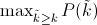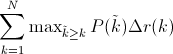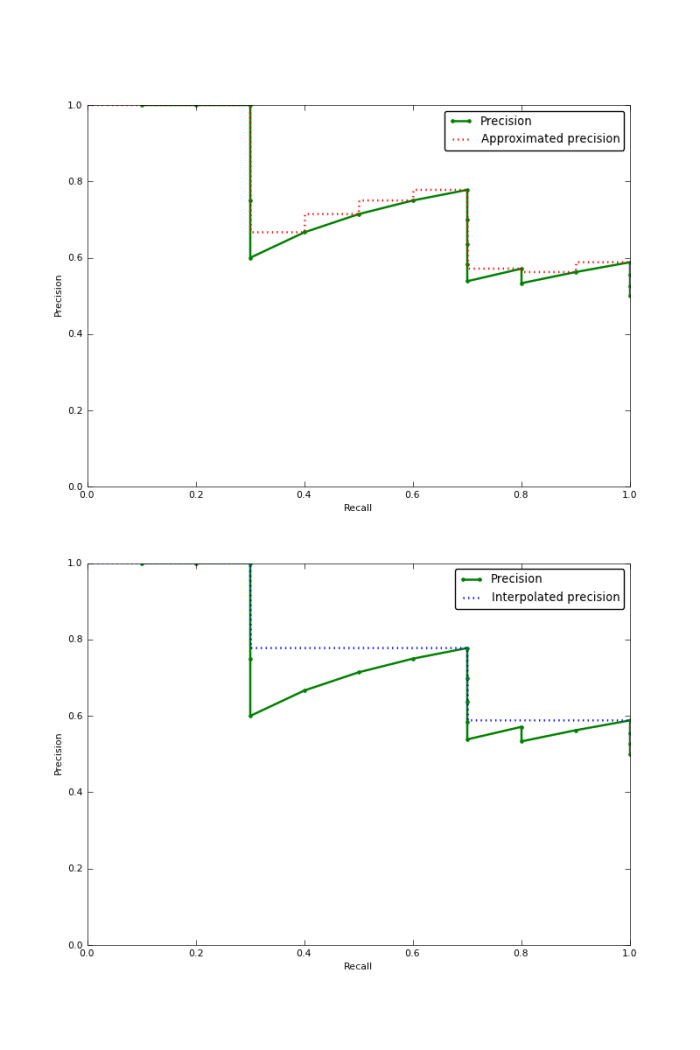## IoU

IoU这一值，可以理解为系统预测出来的框与原来图片中标记的框的重合程度。

IoU=DetectionResultGroundTruthDetectionResultGroundTruth